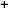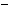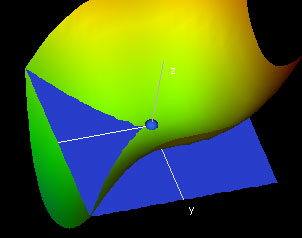Labware - MA35 Multivariable Calculus - Three Variable Calculus
 MA35 Labs 3 » Three Variable Calculus Contents3.2 Differentiation 3.2.1 Partial Derivatives 3.2.2 Critical Points 3.2.3 Tangent Hyperplanes and Normal Vectors 3.2.4 Chain Rule 3.2.5 Differentiability 3.4 Integration Search

Tangent Hyperplanes and Normal Vectors

Text

Consider a point P=(x0,y0,z0) in the domain of f(x,y,z). If at this point the xy-, yz-, and xz-slice curves are differentiable, then their tangent lines determine a hyperplane consisting of the points (x0,y0,z0,f(x0,y0,z0)) that is tangent to the hypersurface at P.This hyperplane is defined as the tangent hyperplane to P and has the equation

w = fx(x0,y0,z0)(x - x0) + fy(x0,y0,z0)(y - y0) + fz(x0,y0,z0)(z - z0) + f(x0,y0,z0).Demos

 Slicing the Tangent HyperplaneIn this demo, we show some slices of the tangent hyperplane at a point P on a function graph of three variables. We slice the tangent plane in the direction of the coordinate axes at the hotspot P (in blue), which you can move around in the Domain-Window. In the Slices-Window you can see the colored slice surfaces of the function graph at the point . As usual, the color of the slice corresponds to the value of f(x,y,z). The slice surfaces are then displayed as function graphs in the XY-, YZ- and XZ- Slices Windows, together with the slices of the tangent hyperplane. Note that the slices of the tangent hyperplane are the tangent planes of the slices(Why?).Exercises

• 1. Find equations for the tangent hyperplanes at the point (x0, y0, z0) for each of the following functions:
• f(x, y, z) = x2 + y2 + z2
• f(x, y, z) = x + y
• f(x, y, z) = xy + 2xz + 3yz
• f(x, y, z) = ex + 2y + sin(z)
• 2. Describe the xy, xz, and yz slices of the tangent hyperplane at a critical point of a function.